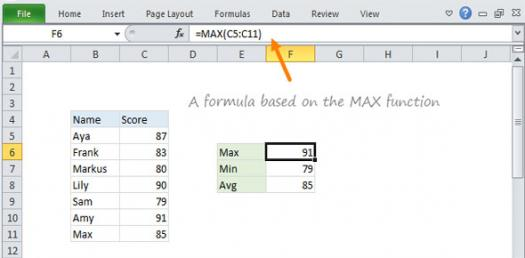# Excel Functions Quiz

Approved & Edited by ProProfs Editorial Team
The editorial team at ProProfs Quizzes consists of a select group of subject experts, trivia writers, and quiz masters who have authored over 10,000 quizzes taken by more than 100 million users. This team includes our in-house seasoned quiz moderators and subject matter experts. Our editorial experts, spread across the world, are rigorously trained using our comprehensive guidelines to ensure that you receive the highest quality quizzes.
| By Calderon_jorge
C
Calderon_jorge
Community Contributor
Quizzes Created: 1 | Total Attempts: 1,056
Questions: 9 | Attempts: 1,060SettingsThis is a review about lesson 3, the topics contained are this: excel formulas, functions( sum,max,min,average,round,if)

• 1.

### To start a formula you must use:

• A.

Parentheses ()

• B.

Equals =

• C.

B. Equals =
Explanation
The correct answer is "Equals =". In order to start a formula, you must use the equals sign (=). This is because the equals sign is used to indicate that you are entering a formula and want the spreadsheet software to perform calculations based on that formula. Without the equals sign, the software will treat the entry as a regular text or number. The parentheses () are used to group certain parts of the formula together, while "Just type your formula" is not a valid way to start a formula.

Rate this question:

• 2.

### Which of the following is invalid?

• A.

=MAX(B5:B15)

• B.

=MAX(B1,B2,B3,B4,B5,B6,B7)=MAX(B8,B9)

• C.

=MAX(B1:B10,B11:B20)

B. =MAX(B1,B2,B3,B4,B5,B6,B7)=MAX(B8,B9)
Explanation
The given answer "=MAX(B1,B2,B3,B4,B5,B6,B7)=MAX(B8,B9)" is invalid because the MAX function can only accept a range of cells or individual cell references as arguments, not multiple separate arguments. Therefore, it is not possible to compare two separate MAX functions using the "=" operator.

Rate this question:

• 3.

• 4.

• 5.

• 6.

• 7.

### You can refer data in worksheet from another?

• A.

True

• B.

False

A. True
Explanation
Yes, you can refer data in a worksheet from another worksheet in Excel. This can be done by using formulas or functions that reference the cell or range of cells in the other worksheet. By linking the data between worksheets, any changes made in the referenced cells will automatically update in the referring worksheet.

Rate this question:

• 8.

• A.

True

• B.

False